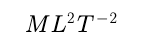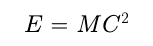# What is Energy? Work, Energy and Power.

Energy

• The energy of a body is defined as its capacity for doing work.
• Since energy of a body is the total quantity of work done therefore it is a scalar quantity.
• Dimension• Mass energy equivalence : Einstein’s special theory of relativity shows that material particle itself is a form of energy. The relation between the mass of a particle m and its equivalent energy is given as
•Examples :

• Annihilation of matter when an electron  and a positron combine with each other, they annihilate or destroy each other. The masses of electron and positron are converted into energy. This energy is released in the form of -gamma rays.

• Nuclear bomb : When the nucleus is split up due to mass defect (The difference in the mass of nucleons and the nucleus) energy is released in the form of gamma-radiations  and heat.

• Various forms of energy
1. Mechanical energy
2. (Kinetic and Potential)
3. Chemical energy
4. Electrical energy
5. Magnetic energy
6. Nuclear energy
7. Sound energy
8. Light energy
9. Heat energy

Transformation of energy    :